# Python Snippets Blog

cancel
Showing results for
Show  only  | Search instead for
Did you mean:

(1 Posts)

## Calculating Zonal Statistics with Python (rasterstats)Occasional Contributor III

These days, it is quite common for people to use the rasterio, rasterstats, numpy, or geopandas Python packages in their Raster processing/analysis workflows. This post aims to illustrate how some of these packages might be used to perform zonal statistics:

• rasterio
• rasterstats
• geopandas
• fiona

If you're ever used the Summarize Raster Within or Zonal Statistics tools and you've wondered a bit about what goes on behind the scenes his article will help break down a few of the processes.

Prerequisites:
A number of packages are required for the below script to work - some of these packages are dependent on gdal.

The easiest way to get started is to use Anaconda to create a new environment:

conda create -n raster python=3.7

conda install -n raster gdal

From there, you can use conda/pip to install the remainder of the packages:

• rasterio
• rasterstats
• descartes
• mapclassify
• geopandas
• fiona
• shapely
• matplotlib

Overview:
When you run a tool like Zonal Statistics or Summarize Raster Within, you are asked for a few things:

1. Input Zone Layer
2. Zone Field
3. Input Raster Layer to Summarize
4. Statistic Type

As an example, let's say you're working with this then:

1. A polygon feature class of parcels
2. Owner Name field
3. DEM
4. Average

In the equivalent workflow presented here, the following occurs: The polygon feature class is loaded into data frame > dissolved by the zone field >  > statistics are calculated using the zones > the result is rasterized.

Begin by importing the required packages:

``import fiona, rasterioimport geopandas as gpdfrom rasterio.plot import showimport matplotlib.pyplot as pltimport rasterio.plot as rpltfrom rasterio.features import rasterizefrom rasterstats import zonal_stats‍‍‍‍‍‍‍‍‍‍‍‍‍‍‍‍‍‍‍‍‍``

In order to run the required tools, it helps to view the data - the below help with adding a bit of interactivity:

``def enum_items(source):    print("\n")    for ele in enumerate(source):         print(ele) def list_columns(df):    field_list = list(df)    enum_items(field_list)    return field_list‍‍‍‍‍‍‍‍‍‍‍‍‍‍‍‍‍‍‍‍‍‍‍‍‍‍‍``

These functions are used to load data into a geopandas dataframe for easy manipulation. Either an .shp or a File Geodatabase Feature class (the input vector layer).

``# For loading feature classes into geopandas dataframedef loadfc_as_gpd(fgdb):    layers = fiona.listlayers(fgdb)    enum_items(layers)    index = int(input("Which index to load? "))    fcgpd = gpd.read_file(fgdb,layer=layers[index])    return fcgpd # For loading shapefiles into geopandas dataframedef loadshp_as_gpd(shp):    data = gpd.read_file(shp)    return data‍‍‍‍‍‍‍‍‍‍‍‍‍‍‍‍‍‍‍‍‍‍‍‍‍‍‍‍‍‍‍‍‍‍‍‍``

If you want to filter the data before processing (e.g., maybe you only want to use parcels within a certain county):

``# For optional filtering of datadef filter_gpd(fcgpd):    field_list = list_columns(fcgpd)    index = int(input("Filter by which field (index)? "))    field = field_list[index]    value = input("Enter value: ")    result = fcgpd[fcgpd[field] == value]    return result‍‍‍‍‍‍‍‍‍‍‍‍‍‍‍‍‍‍‍‍‍‍‍‍``

Ideally, the input vector layer is in the same projection as the input raster layer. If it's not, this function can be used to re-project the vector layer to the raster's projection. The re-projected vector layer is plotted along with the raster data for visual inspection:

``# For re-projecting input vector layer to raster projectiondef reproject(fcgpd, raster):    proj = raster.crs.to_proj4()    print("Original vector layer projection: ", fcgpd.crs)    reproj = fcgpd.to_crs(proj)    print("New vector layer projection (PROJ4): ", reproj.crs)    fig, ax = plt.subplots(figsize=(15, 15))    rplt.show(raster, ax=ax)    reproj.plot(ax=ax, facecolor='none', edgecolor='red')    fig.show()    return reproj‍‍‍‍‍‍‍‍‍‍‍‍‍‍‍‍‍‍‍‍‍‍‍‍‍‍‍‍‍‍‍‍‍‍‍‍‍‍‍‍‍‍‍‍``
• Note: the to_proj4 method is introduced in later versions of rasterio (e.g. 1.0.22).

This is used to perform a dissolve on the selected zone field:

``# For dissolving geopandas dataframe by selected fielddef dissolve_gpd(df):    field_list = list_columns(df)    index = int(input("Dissolve by which field (index)? "))    dgpd = df.dissolve(by=field_list[index])    return dgpd‍‍‍‍‍‍‍‍‍‍‍‍‍‍‍‍‍‍‍‍‍‍‍‍``

Here desired statistics are selected - I've included a list of common statistics but rasterstats offers additional options. You can actually select multiple statistics to calculate and this may be one reason why someone would choose to incorporate a tool like rasterstats in their workflow (Zonal Statistics as Table will let you get multiple statistics, but not Zonal Statistics/Summarize Raster Within😞

``# For selecting which raster statistics to calculatedef stats_select():    stats_list = ['min', 'max', 'mean', 'count',               'sum', 'std', 'median', 'majority',               'minority', 'unique', 'range']    enum_items(stats_list)    indices = input("Enter raster statistics selections separated by space: ")    stats  = list(indices.split())    out_stats = list()    for i in stats:        out_stats.append(stats_list[int(i)])    return out_stats # For calculating zonal statisticsdef get_zonal_stats(vector, raster, stats):    # Run zonal statistics, store result in geopandas dataframe    result = zonal_stats(vector, raster, stats=stats, geojson_out=True)    geostats = gpd.GeoDataFrame.from_features(result)    return geostats‍‍‍‍‍‍‍‍‍‍‍‍‍‍‍‍‍‍‍‍‍‍‍‍‍‍‍‍‍‍‍‍‍‍‍‍‍‍‍‍‍‍‍‍‍‍‍‍‍‍‍‍‍‍‍‍‍``

The result of rasterstats' zonal_stats function is not a raster (e.g. .tif) by default so you must rasterize the result. In the previous function, the result is stored as GeoJSON in a geopandas dataframe and this is what gets rasterized to a .tif.

``# For generating raster from zonal statistics resultdef stats_to_raster(zdf, raster, stats, out_raster, no_data='y'):    meta = raster.meta.copy()    out_shape = raster.shape    transform = raster.transform    dtype = raster.dtypes    field_list = list_columns(stats)    index = int(input("Rasterize by which field? "))    zone = zdf[field_list[index]]    shapes = ((geom,value) for geom, value in zip(zdf.geometry, zone))    burned = rasterize(shapes=shapes, fill=0, out_shape=out_shape, transform=transform)    show(burned)    meta.update(dtype=rasterio.float32, nodata=0)    # Optional to set nodata values to min of stat    if no_data == 'y':        cutoff = min(zone.values)        print("Setting nodata cutoff to: ", cutoff)        burned[burned < cutoff] = 0     with rasterio.open(out_raster, 'w', **meta) as out:        out.write_band(1, burned)    print("Zonal Statistics Raster generated")‍‍‍‍‍‍‍‍‍‍‍‍‍‍‍‍‍‍‍‍‍‍‍‍‍‍‍‍‍‍‍‍‍‍‍‍‍‍‍‍‍‍‍‍‍‍‍‍‍‍‍‍‍‍‍‍‍‍‍‍‍‍‍``

Altogether the script is:

``import fiona, rasterioimport geopandas as gpdfrom rasterio.plot import showimport matplotlib.pyplot as pltimport rasterio.plot as rpltfrom rasterio.features import rasterizefrom rasterstats import zonal_statsdef enum_items(source):    print("\n")    for ele in enumerate(source):         print(ele)def list_columns(df):    field_list = list(df)    enum_items(field_list)    return field_list# For loading feature classes into geopandas dataframedef loadfc_as_gpd(fgdb):    layers = fiona.listlayers(fgdb)    enum_items(layers)    index = int(input("Which index to load? "))    fcgpd = gpd.read_file(fgdb,layer=layers[index])    return fcgpd# For loading shapefiles into geopandas dataframedef loadshp_as_gpd(shp):    data = gpd.read_file(shp)    return data# For optional filtering of datadef filter_gpd(fcgpd):    field_list = list_columns(fcgpd)    index = int(input("Filter by which field (index)? "))    field = field_list[index]    value = input("Enter value: ")    result = fcgpd[fcgpd[field] == value]    return result# For re-projecting input vector layer to raster projection (Rasterio v. 1.0.22)def reproject(fcgpd, raster):    proj = raster.crs.to_proj4()    print("Original vector layer projection: ", fcgpd.crs)    reproj = fcgpd.to_crs(proj)    print("New vector layer projection (PROJ4): ", reproj.crs)    fig, ax = plt.subplots(figsize=(15, 15))    rplt.show(raster, ax=ax)    reproj.plot(ax=ax, facecolor='none', edgecolor='red')    fig.show()    return reproj# For dissolving geopandas dataframe by selected fielddef dissolve_gpd(df):    field_list = list_columns(df)    index = int(input("Dissolve by which field (index)? "))    dgpd = df.dissolve(by=field_list[index])    return dgpd# For selecting which raster statistics to calculatedef stats_select():    stats_list = ['min', 'max', 'mean', 'count',               'sum', 'std', 'median', 'majority',               'minority', 'unique', 'range']    enum_items(stats_list)    indices = input("Enter raster statistics selections separated by space: ")    stats  = list(indices.split())    out_stats = list()    for i in stats:        out_stats.append(stats_list[int(i)])    return out_stats# For calculating zonal statisticsdef get_zonal_stats(vector, raster, stats):    # Run zonal statistics, store result in geopandas dataframe    result = zonal_stats(vector, raster, stats=stats, geojson_out=True)    geostats = gpd.GeoDataFrame.from_features(result)    return geostats# For generating raster from zonal statistics resultdef stats_to_raster(zdf, raster, stats, out_raster, no_data='y'):    meta = raster.meta.copy()    out_shape = raster.shape    transform = raster.transform    dtype = raster.dtypes    field_list = list_columns(stats)    index = int(input("Rasterize by which field? "))    zone = zdf[field_list[index]]    shapes = ((geom,value) for geom, value in zip(zdf.geometry, zone))    burned = rasterize(shapes=shapes, fill=0, out_shape=out_shape, transform=transform)    show(burned)    meta.update(dtype=rasterio.float32, nodata=0)    # Optional to set nodata values to min of stat    if no_data == 'y':        cutoff = min(zone.values)        print("Setting nodata cutoff to: ", cutoff)        burned[burned < cutoff] = 0     with rasterio.open(out_raster, 'w', **meta) as out:        out.write_band(1, burned)    print("Zonal Statistics Raster generated")def main():    rst = '/path/to.raster.tif'    raster = rasterio.open(rst)    fgdb = '/path/to/fgdb.gdb'    vector = loadfc_as_gpd(fgdb)        p_vector = reproject(vector, raster)        d_vector = dissolve_gpd(p_vector)    out_rst = '/path/to/out.tif'    stats_to_get = stats_select()    zs = get_zonal_stats(d_vector, rst, stats_to_get)    stats_to_raster(zs, raster, stats_to_get, out_rst)if __name__ == '__main__':    main()‍‍‍‍‍‍‍‍‍‍‍‍‍‍‍‍‍‍‍‍‍‍‍‍‍‍‍‍‍‍‍‍‍‍‍‍‍‍‍‍‍‍‍‍‍‍‍‍‍‍‍‍‍‍‍‍‍‍‍‍‍‍‍‍‍‍‍‍‍‍‍‍‍‍‍‍‍‍‍‍‍‍‍‍‍‍‍‍‍‍‍‍‍‍‍‍‍‍‍‍‍‍‍‍‍‍‍‍‍‍‍‍‍‍‍‍‍‍‍‍‍‍‍‍‍‍‍‍‍‍‍‍‍‍‍‍‍‍‍‍‍‍‍‍‍‍‍‍‍‍‍‍‍‍‍‍‍‍‍‍‍‍‍‍‍‍‍‍‍‍‍‍‍‍‍‍‍‍‍‍‍‍‍‍‍‍‍‍‍‍‍‍‍‍‍‍‍‍‍‍‍‍‍‍‍‍‍‍‍‍‍‍‍‍‍‍‍‍‍‍‍‍‍‍‍‍‍‍‍‍‍‍‍‍‍‍‍‍‍‍‍‍‍‍‍‍‍‍‍‍‍‍‍‍‍‍‍‍‍‍‍‍‍‍‍‍‍‍‍‍‍‍‍‍‍‍‍‍‍‍‍‍‍‍‍‍‍‍‍‍‍‍‍‍‍‍‍‍‍‍‍‍‍‍‍‍‍‍‍‍‍‍‍‍‍‍‍‍‍‍‍‍‍‍‍‍‍‍‍‍‍‍‍‍‍‍‍‍‍‍‍‍‍‍‍‍‍‍‍‍‍‍‍‍‍‍‍‍‍‍‍‍‍‍‍‍‍‍‍‍‍‍‍‍‍‍‍‍‍‍‍‍‍‍‍‍‍‍‍‍‍‍‍‍‍‍‍‍‍‍‍‍‍‍‍‍‍‍‍‍‍‍‍‍‍‍‍‍‍‍‍‍‍‍‍‍‍‍‍‍‍‍‍‍‍‍‍‍‍‍‍‍‍‍‍‍‍‍‍‍‍‍‍‍‍‍‍‍‍‍‍‍‍‍‍‍‍‍‍‍‍‍‍‍‍‍‍‍‍‍‍‍``

Usage:

As an example, suppose we have the following data where the vector layer is a feature class of of urban areas and the raster layer is a DEM: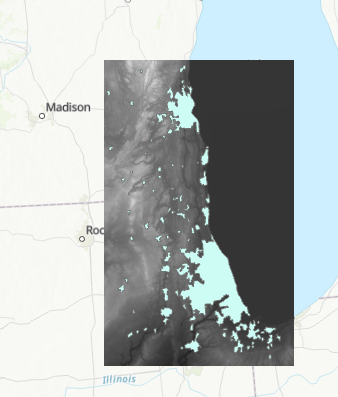Start by setting the paths in the main function of the final script then run either in a terminal or a notebook (I ran it in a notebook). The first prompt will ask you which Feature Class in the File Geodatabase you would like to load. Enter the index:From review of the data, I know the vector layer needs to be projected so I included the reproject function in main. Here you can see that the data was re-projected to North America Albers Equal Area Conic (the proj4 representation of this projection is shown):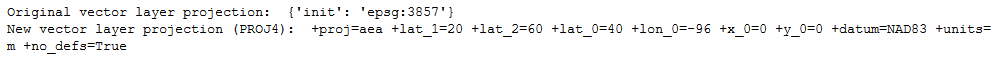The result is plotted for visual inspection:Next, a prompt to choose which field to dissolve on: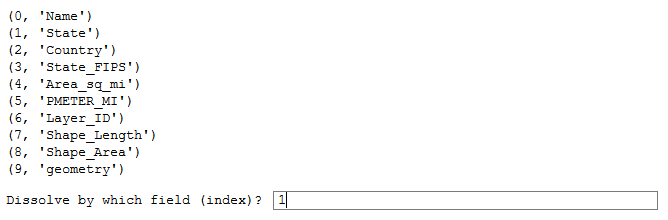Here I select the statistics I want to calculate, separating the selections by space: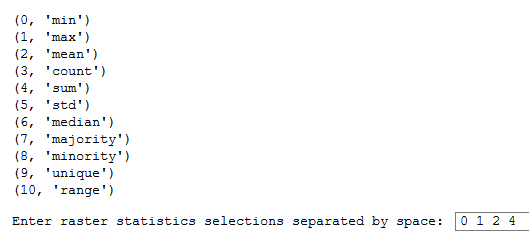Finally, the statistic to use for rasterization is selected: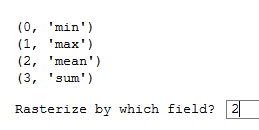The result is plotted:This compares well with the result you would get from the Zonal Statistics tool: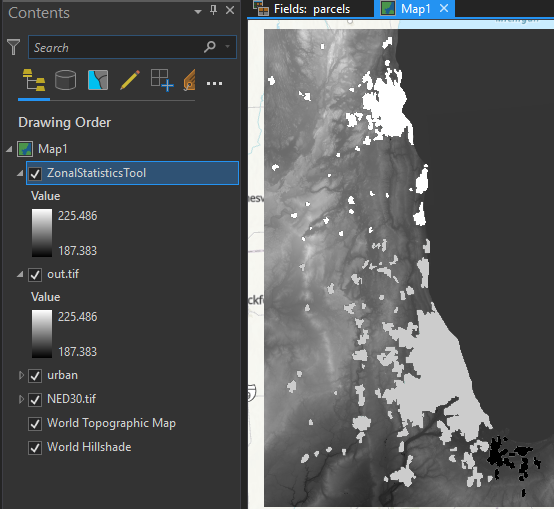more
4 0 7,272